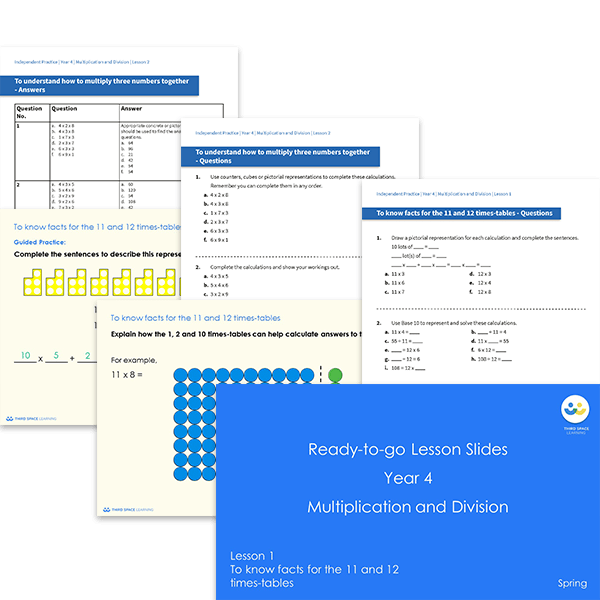# Ready-to-go lessons Year 4 Multiplication and Division (Spring Block 1) Slides and Worksheets

Multiplication and division ready-to-go lessons for Year 4 includes 11 PowerPoints with matching worksheets ready for you to use with a class. These resources follow a mastery approach to mathematics teaching, similar to schemes such as White Rose Maths and Mathematics Mastery. The PowerPoints are fully editable with detailed notes for every teaching slide. Each associated worksheet is divided into three parts with a range of activities to complete across the block.

This Year 4 Multiplication and Division pack contains 11 fully editable PowerPoints and 11 worksheets.

The slides and worksheets cover:

• The 11 and 12 times tables
• Multiplying three numbers
• Understanding and identifying factor pairs
• Using different methods to complete calculations
• Multiplying a 2-digit number by a 1-digit number and multiplying a 3-digit number by a 1-digit number
• Dividing a 2-digit number by a 1-digit number (including with remainders)  and dividing a 3-digit number by a 1-digit number
• Solving correspondence problems

Year 4

Multiplication, Division